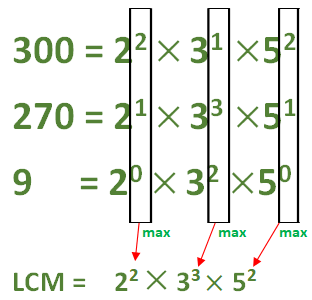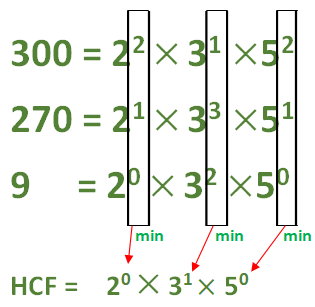Related Articles

# Finding the LCM and HCF of decimal numbers

• Last Updated : 12 Aug, 2020

Finding the LCM and HCF are most frequently asked questions in any competitive exams. Finding LCM and HCF for natural numbers are taught in our school level but in some exams they also ask to find the LCM and HCF of decimal numbers.

There is easy process to find the LCM and HCF of decimal numbers similar to natural numbers with some changes.

Example-1:
Find the HCF and LCM of 3, 2.7, 0.09

Explanation:

• Step-1:
Write all the numbers with same number of digits after decimal point.

`3.00, 2.70, 0.09 `
• Step-2:
Now count the number of digits after decimal point (value is 2 for above problem) and calculate 10 power of the obtained value. Let the number be n = 102 = 100.
• Step-3:
Now remove the decimal point and find the LCM and HCF of the numbers.
```LCM(300, 270, 9) and HCF(300, 270, 9).

300 = 22 x 31 x 52

270 = 21 x 33 x 51

9 = 20 x 32 x 50

LCM(300, 270, 9) = 22 x 33 x 52 = 2700

HCF(300, 270, 9) = 20 x 31 x 50 = 3 ```

For finding the LCM and HCF, we should write the number in the power of prime numbers as written above. We should ensure that all the numbers should be written as power of prime numbers of same number. Example : 9 can be written as 32 but the other two numbers also contain 2 and 5 as primes. So we can write other two numbers as powers of 0. So 9 can be written as 20 x 32 x 50 and it won’t change the value of the number.

Since we have our numbers in form of power of primes, Now the LCM is the number formed as the product of primes with its power is maximum value of the power of the same prime in given numbers.```LCM = 2 power of max(2, 1, 0) x 3 power of max(1, 3, 2) x 5 power of max(2, 1, 0)
= 22 x 33 x 52 = 2700 ```

HCF calculation is similar but with only one change. Instead of taking max in power we take min in power.```HCF = 2 power of min(2, 1, 0) x 3 power of min(1, 3, 2) x 5 power of min(2, 1, 0)
= 20 x 31 x 50 = 3 ```
• Step-4:
Now divide the obtained answer with our number n in step 2. The value we obtain is our required answer.

```LCM(3, 2.7, 9) = 2700/100 = 27

HCF(3, 2.7, 9) = 3/100 = 0.03
```

Example-2:
Find LCM and HCF of 0.216, 6, 2.

Explanation:
By using the above steps, in similar way we can do this problem.

• Step-1:
`0.216, 6, 2 -> 0.216, 6.000, 2.000 `
• Step-2:
Since the number of digits after decimal point is 3, the value of n = 103 = 1000.
• Step-3:
Find LCM (216, 6000, 2000) and HCF (216, 600, 200).
```216 = 23 x 33 = 23 x 33 x 50

6000 = 24 x 31 x 53 = 24 x 31 x 53

2000 = 24 x 53 = 24 x 30 x 53

LCM(216, 600, 200) = 24 x 33 x 53 = 54000

HCF(216, 600, 200) = 23 x 30 x 50 = 8
```
• Step-4:
```LCM(0.216, 6, 2) = 5400/1000 = 54

HCF(0.216, 6, 2) = 8/1000 = 0.008 ```

Example-3:
Find the LCM and HCF of 0.63, 1.05.

Explanation :

• Step-1:
`0.63, 1.05 -> 0.63, 1.05 `
• Step-2:
Since the number of digits after decimal point is 2, the value of n = 102 = 100.
• Step-3:
Remove decimal point and find LCM (63, 105) and HCF(63, 105).
```63 = 32 x 50 x 71

105 = 31 x 51 x 71

LCM (63, 105) = 32 x 51 x 71 = 315

HCF(63, 105) = 31 x 50 x 71 = 21
```
• Step-4:
```LCM (0.63, 1.05) = 315/100 = 3.15

HCF(0.63, 1.05) = 21/100 = 2.1
```

In this simple way we can find the LCM and HCF of any number of decimals of any number.

Attention reader! Don’t stop learning now.  Practice GATE exam well before the actual exam with the subject-wise and overall quizzes available in GATE Test Series Course.

Learn all GATE CS concepts with Free Live Classes on our youtube channel.

My Personal Notes arrow_drop_up